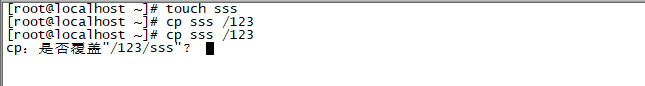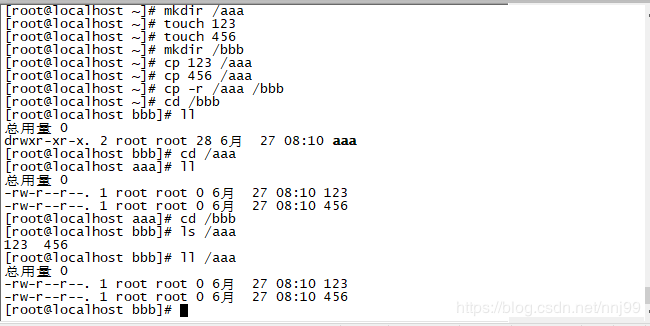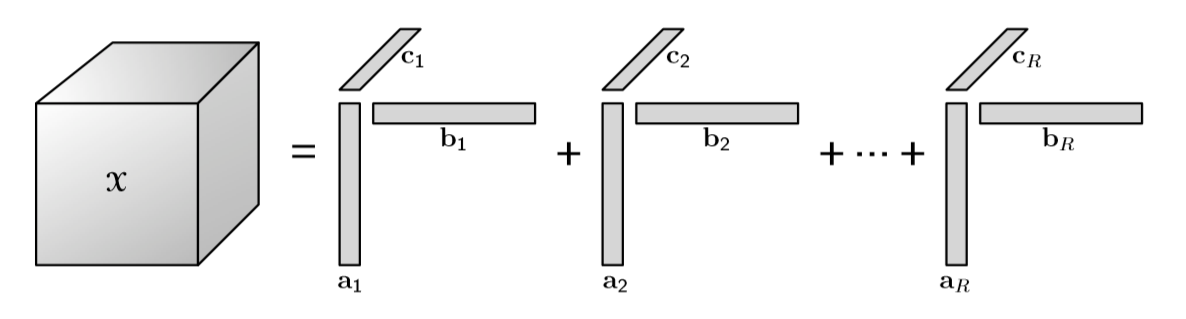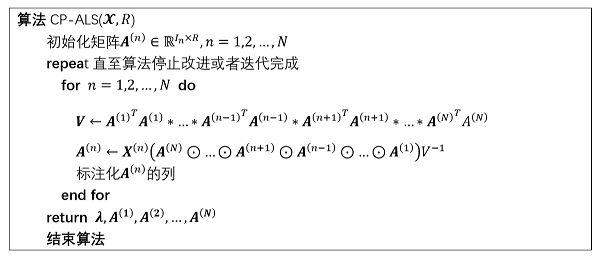• jetson 安装pytorch1.7版本，免费供大家下载，安装方法请查看https://blog.csdn.net/u011119817/article/details/109616474
• tensorflow-2.1.0-cp36-cp36m-win_amd64.whltf
• PyQt5，安装方法：1.打开cmd 2.安装whl文件，PyQt5-5.11.2-5.11.1-cp35.cp36.cp37.cp38-none-win_amd64.whl
• pygame.whl -1.9.3-cp37-cp37m-win_amd64+win32 适用于最新的Python 3.7
• cp2104的老驱动不支持win10，折腾了好一会儿，网上的很多连接都是假的，本资源亲测可用。下载紧要5分。之前不要分的，没办法，我也得赚点分。
• 2018.09.02 【小白】openCV_python入门杂记（1... 最后找到了opencv_python‑3.4.3‑cp37‑cp37m‑win_amd64.whl的资源，在此进行分享，希望对各位有帮助。 【PS：本来想免费上传的，不过貌似现在只能最低1分上传了】
• CP210X，CP2102 USB转串口驱动，完美支持win7 win8 win10
• cp2102驱动亲测win10可用,直接安装，快速上手。。。。。。。。。。。。。。。。。。。。
• whl文件，dlib-19.3.1-cp35-cp35m-win_amd64.whl ，dlib-19.3.1-cp35-cp35m-win_amd64.whl
• 64位Python36，pycrypto-2.6.1-cp36-cp36m-win_amd64.whl
• opencv_python-3.4.1-cp35-cp35m-win_amd64.whl，windows环境+python3，pip安装不成功的可以手动安装
• dlib库的whl文件——dlib-19.7.0-cp36-cp36m-win_amd64.rar 。dlib库的whl文件——dlib-19.7.0-cp36-cp36m-win_amd64.rardlib whl
• silicon_labscp210x 转串口驱动，包括32位，64位驱动，win10下测试完美兼容，网上不是找不到，只是比较麻烦！
• ## cp命令

万次阅读 多人点赞 2019-07-01 14:20:22
cp 命令，主要用来复制文件和目录，同时借助某些选项，还可以实现复制整个目录，以及比对两文件的新旧而予以升级等功能。 cp 命令的基本格式如下： [root@localhost ~]# cp [选项] 源文件 目标文件 选项： -a：相当...
cp 命令，主要用来复制文件和目录，同时借助某些选项，还可以实现复制整个目录，以及比对两文件的新旧而予以升级等功能。
cp 命令的基本格式如下：
[root@localhost ~]# cp [选项] 源文件 目标文件

选项： -a：相当于 -d、-p、-r 选项的集合，这几个选项我们一一介绍； -d：如果源文件为软链接（对硬链接无效），则复制出的目标文件也为软链接； -i：询问，如果目标文件已经存在，则会询问是否覆盖； -l：把目标文件建立为源文件的硬链接文件，而不是复制源文件； -s：把目标文件建立为源文件的软链接文件，而不是复制源文件； -p：复制后目标文件保留源文件的属性（包括所有者、所属组、权限和时间）； -r：递归复制，用于复制目录； -u：若目标文件比源文件有差异，则使用该选项可以更新目标文件，此选项可用于对文件的升级和备用。
需要注意的是，源文件可以有多个，但这种情况下，目标文件必须是目录才可以。 这里的软链接，类似于 Windows 系统中的快捷方式，而硬链接则是透过文件系统的 inode 号产生一个新的文件名。无论是复制软链接还是硬链接，都不是复制源文件。有关软链接和硬链接更详细的介绍，可阅读《Linux ln命令》一节。
【例 1】cp 命令基本用法 cp 命令既可以复制文件，也可以复制目录。我们先来看看如何复制文件，例如：
[root@localhost ~]# touch cangls
#建立源文件
[root@localhost ~]# cp cangls /tmp/
#把源文件不改名复制到 /tmp/ 目录下

如果需要改名复制，则命令如下：
[root@localhost ~]# cp cangls /tmp/bols
#改名复制

如果复制的目标位置已经存在同名的文件，则会提示是否覆盖，因为 cp 命令默认执行的是“cp -i”的别名，例如：
[root@localhost ~]# cp cangls /tmp/
cp:是否覆盖"/tmp/cangls"?y
#目标位置有同名文件，所以会提示是否覆盖

接下来我们看看如何复制目录，其实复制目录只需使用“-r”选项即可，例如：
root@localhost ~]# mkdir movie
#建立测试目录
[root@localhost ~]# cp -r /root/movie/ /tmp/
#目录原名复制

【例 2】复制软链接文件 如果源文件不是一个普通文件，而是一个软链接文件，那么是否可以复制软链接的属性呢？我们试试：
[root@localhost ~]# ln -s /root/cangls /tmp/cangls_slink
lrwxrwxrwx 1 root root 12 6 月 14 05:53 /tmp/cangls_slink -> /root/cangls
#源文件本身就是一个软链接文件
#复制软链接文件，但是不加"-d"选项
[root@localhost ~]# cp -d /tmp/cangls_slink /tmp/cangls_t2
#复制软链接文件，加入"-d"选项
[root@localhost ~]# ll /tmp/cangls_t1 /tmp/cangls_t2
-rw-r--r-- 1 root root 0 6月 14 05:56 /tmp/cangls_t1
#会发现不加"-d"选项，实际复制的是软链接的源文件，而不是软链接文件
lrwxrwxrwx 1 root root 12 6 月 14 05:56/tmp/ cangls_t2-> /root/cangls
#而如果加入了"-d"选项，则会复制软链接文件

这个例子说明，如果在复制软链接文件时不使用 “-d” 选项，则 cp 命令复制的是源文件，而不是软链接文件；只有加入了 “-d” 选项，才会复制软链接文件。请大家注意，"-d" 选项对硬链接是无效的。
【例 3】保留源文件属性复制 我们发现，在执行复制命令后，目标文件的时间会变成复制命令的执行时间，而不是源文件的时间。例如：

[root@localhost ~]# cp /var/lib/mlocate/mlocate.db /tmp/
[root@localhost ~]# ll /var/lib/mlocate/mlocate.db
-rw-r-----1 root slocate2328027 6月 14 02:08/var/lib/mlocate/mlocate.db
#注意源文件的时间和所属组
[root@localhost ~]#ll /tmp/mlocate.db
-rw-r----- 1 root root2328027 6 月 14 06:05/tmp/mlocate.db
#由于复制命令由root用户执行，所以目标文件的所属组为了root，而且时间也变成了复制命令的执行时间

而当我们执行备份、曰志备份的时候，这些文件的时间可能是一个重要的参数，这就需执行 “-p” 选项了。这个选项会保留源文件的属性，包括所有者、所属组和时间。例如：
[root@localhost ~]# cp -p /var/lib/mlocate/mlocate.db /tmp/mlocate.db_2
#使用"-p"选项
[root@localhost ~]# ll /var/lib/mlocate/mlocate.db /tmp/mlocate.db_2
-rw-r----- root slocate 2328027 6月 14 02:08 /tmp/mlocate.db_2
-rw-r----- root slocate 2328027 6月 14 02:08 /var/lib/mlocate/mlocate.db
#源文件和目标文件的所有属性都一致，包括时间

我们之前讲过，"-a" 选项相当于 “-d、-p、-r” 选项，这几个选项我们已经分别讲过了。所以，当我们使用 “-a” 选项时，目标文件和源文件的所有属性都一致，包括源文件的所有者，所属组、时间和软链接性。使用 “-a” 选项来取代 “-d、-p、-r” 选项更加方便。
【例 4】 “-l” 和 “-s” 选项 我们如果使用 “-l” 选项，则目标文件会被建立为源文件的硬链接；而如果使用了 “-s” 选项，则目标文件会被建立为源文件的软链接。
这两个选项和 “-d” 选项是不同的，“d” 选项要求源文件必须是软链接，目标文件才会复制为软链接；而 “-l” 和 “-s” 选项的源文件只需是普通文件，目标文件就可以直接复制为硬链接和软链接。例如：
[root@localhost ~]# touch bols
#建立测试文件
[root@localhost ~]# ll -i bols
262154-rw-r--r-- 1 root root 0 6月 14 06:26 bols
#源文件只是一个普通文件，而不是软链接文件
[root@localhost ~]# cp -l /root/bols /tmp/bols_h
[root@localhost ~]# cp -s /root/bols /tmp/bols_s
#使用"-l" 和"-s"选项复制
[root@localhost ~]# ll -i /tmp/bols_h /tmp/bols_s
262154-rw-r--r-- 2root root 0 6 月 14 06:26/tmp/bols_h
#目标文件 /tmp/bols_h 为源文件的硬链接文件
932113 lrwxrwxrwx 1 root root 10 6 月 14 06:27/tmp/bols_s -> /root/bols
#目标文件 /tmp/bols_s 为源文件的软链接文件

展开全文• CP210x_VCP_Win7.zip　CP2102驱动64位win10可用，CP2102驱动64位win10可用，CP2102驱动64位win10可用
• ## cp

千次阅读 2019-07-15 08:38:15
cp 复制文件或目录命令 cp 命令的基本格式如下： [root@localhost ~]# cp [选项] 源文件 目标文件 1. cp 命令默认执行的是“cp -i”的别名 我们来看如何复制文件： 如图，我在家目录里面建立了一个源文件(空文件) ...
cp
复制文件或目录命令 cp 命令的基本格式如下：
[root@localhost ~]# cp [选项] 源文件 目标文件

1. cp 命令默认执行的是“cp -i”的别名 我们来看如何复制文件：如图，我在家目录里面建立了一个源文件(空文件) cangls， 和目录 123 . 把源文件不改名的复制到 123 目录下。
注意：家目录里创建的目录，不能修改文件名并复制放到该目录。 修改文件名并复制到另一个目录下需要在根目录下进行，如图：我在家目录里创建了 “nnn” 文件，又建了一个根目录。 并将该“nnn” 文件改名，复制到根目录 “123” 内。
如果复制的目标位置已经存在同名的文件，则会提示是否覆盖。新建一个文件，复制到根目录，如果在复制一遍，它就会提示你是否要覆盖。 “y” 覆盖。 “n” 不覆盖.
2. cp -r 复制目录到另一个目录下，如图：创建了两个根目录，两个文件(123和456)，将文件都保存在第一个根目录(/aaa)下，可以用(cp -r)命令将(/aaa)目录包括目录下的文件都复制到(/bbb)根目录下。
展开全文• silicon labs 官方驱动，支持Windows 7/8/8.1/10 (v6.7.3) 亲测可用
• lxml-3.6.4-cp35-cp35m-win_amd64.whllxml
• cp2102 usb to uart 驱动，适合64位系统和x86系统
• 在python3.5基础上安装OpenCv库的.whl文件
• 欧姆龙CP1E CP1L CP1H系列PLC USB解密软件
• 这个软件是一个Java实现基于“ciphertext-policy属性加密”(CP-ABE)。　使用该软件,您需要Java加密为基础的配对库(jPBC)安装(jpbc-1.2.0测试)。
• ## CP5611驱动程序

热门讨论 2014-08-17 22:06:21
西门子CP5611通讯卡驱动程序压缩包，需要的请下载
• 施乐彩CP105 CP205 CM205b CM205f维修手册(PDF格式中文版)
• USB转串口驱动 Silicon Labs CP210x USB to UART Bridge VERSION:6.7.2.200 DATE:2015/11/20
• ## linux命令-cp命令

万次阅读 多人点赞 2018-06-21 22:38:00
cp命令 简介 cp（Copy file）：将源文件复制至目标文件 或将多个源文件复制至目标目录。 语法 cp [选项] ... [-T] 源文件 目标文件 cp [选项] ... 源文件 目录 cp [选项] ... -t 目录 源文件 ...
cp命令
简介

cp（Copy file）：将源文件复制至目标文件  或将多个源文件复制至目标目录。

语法
    cp [选项] ... [-T] 源文件 目标文件
cp [选项] ... 源文件  目录
cp [选项] ... -t 目录 源文件
选项
-a， -archive : 等于-dR –preserve=all,与同时指定 -dpR 这三个选项效果一样，用于复制整个目录，包括目录中的子目录等都递归的复制，而且还要保持文件的访问模式，所有者，时间戳等属性与原文件一样。--backup[=CONTROL] : 为每个已存在的目标文件创建备份,类似–backup 但不接受参数-b : 类似–backup 但不接受参数--copy-contents : 在递归(recursive)处理时复制特殊文件内容-d : 等于–no-dereference –preserve=links-f，-force : 如果目标文件无法打开则将其移除并重试(当 -n 选项存在时则不需再选此项)-i，--interactive: 覆盖前询问(使前面的 -n 选项失效)  - 默认cp命令覆盖目标文件时是不会提示的，很多Linux发行版里的cp都被设置别名cp -i,其实作用就是给用户一个提醒。  - 如果你不想被提示，那么请这样输入：\cp source target，或者使用cp命令的绝对路径/bin/cp-H：跟随源文件中的命令行符号链接-l，-link : 对源文件建立硬链接，而非复制文件-L， -dereference ： 总是跟随符号链接-n ，-no-clobber ： 不要覆盖已存在的文件(使前面的 -i 选项失效)-P, --no-dereference : 不跟随源文件中的符号链接-p : 等于–preserve=模式,所有权,时间戳--preserve [=属性列表] 保持指定的属性(默认：模式,所有权,时间戳)，如果可能保持附加属性：环境、链接、xattr 等-c : same as –preserve=context--sno-preserve=属性列表：不保留指定的文件属性--parents : 复制前在目标目录创建来源文件路径中的所有目录-R,-r, --recursive: 递归复制目录及其子目录内的所有内容--reflink[=WHEN] : 控制克隆/CoW 副本。请查看下面的内如。--remove-destination : 尝试打开目标文件前先删除已存在的目的地文件 (相对于 –force 选项)--sparse=WHEN : 控制创建稀疏文件的方式--strip-trailing-slashes : 删除参数中所有源文件/目录末端的斜杠-s, --symbolic-link : 只创建符号链接而不复制文件-S, --suffix=后缀 : 自行指定备份文件的后缀-t, --target-directory=目录 : 将所有参数指定的源文件/目录 复制至目标目录-T, --no-target-directory 将目标目录视作普通文件-u, --update : copy only when the SOURCE file is newer than the destination file or when the destination file is missing-v, --verbose : 详细显示命令执行的操作。-x, --one-file-system 复制的文件或目录存放的文件系统，必须与cp指令执行时所处的文件系统相同，否则不复制，亦不处理位于其他分区的文件-Z, --context=CONTEXT : set security context of copy to CONTEXT
实例
1、将文件a.txt 复制成文件b.txt

[root@VM_0_4_centos cp]# touch a.txt
[root@VM_0_4_centos cp]# cp a.txt b.txt
[root@VM_0_4_centos cp]# ll
total 0
-rw-r--r-- 1 root root 0 Jun 21 19:59 a.txt
-rw-r--r-- 1 root root 0 Jun 21 19:59 b.txt
2、将文件a.txt复制成文件b.txt，显示详细信息

[root@VM_0_4_centos cp]# cp -v a.txt c.txt
‘a.txt’ -> ‘c.txt’
3、复制文件，只有源文件较目的文件的修改时间新时，才复制文件

[root@VM_0_4_centos cp]# cp -uv a.txt d.txt
‘a.txt’ -> ‘d.txt’
[root@VM_0_4_centos cp]# ll
-rw-r--r-- 1 root root 0 Jun 21 19:59 a.txt
-rw-r--r-- 1 root root 0 Jun 21 19:59 b.txt
-rw-r--r-- 1 root root 0 Jun 21 21:46 c.t
-rw-r--r-- 1 root root 0 Jun 21 21:47 c.txt
-rw-r--r-- 1 root root 0 Jun 21 21:48 d.txt

[root@VM_0_4_centos cp]# cp -uv a.txt d.txt
[root@VM_0_4_centos cp]# ll
total 0
-rw-r--r-- 1 root root 0 Jun 21 19:59 a.txt
-rw-r--r-- 1 root root 0 Jun 21 19:59 b.txt
-rw-r--r-- 1 root root 0 Jun 21 21:46 c.t
-rw-r--r-- 1 root root 0 Jun 21 21:47 c.txt
-rw-r--r-- 1 root root 0 Jun 21 21:48 d.txt

4、采用交互方式将文件a.txt复制成文件d.txt

[root@VM_0_4_centos cp]# cp -i  a.txt e.txt
cp: overwrite ‘e.txt’? y
5、将文件a.txt复制成d.txt，因为目的文件已经存在，所以指定使用强制复制的模式

[root@VM_0_4_centos cp]# \cp -f a.txt e.txt
6、递归将目录dir1复制到目录dir2下面（此时dir2不存在）

[root@VM_0_4_centos cp]# cp dir1 dir2
cp: omitting directory ‘dir1’
[root@VM_0_4_centos cp]# cp -r dir1 dir2

7、复制时保留文件属性

[root@VM_0_4_centos cp]# cp -p a.txt f.txt
[root@VM_0_4_centos cp]# ll
total 8
-rw-r--r-- 1 root root    0 Jun 21 19:59 a.txt
-rw-r--r-- 1 root root    0 Jun 21 19:59 b.txt
-rw-r--r-- 1 root root    0 Jun 21 21:46 c.t
-rw-r--r-- 1 root root    0 Jun 21 21:47 c.txt
drwxr-xr-x 2 root root 4096 Jun 21 22:03 dir1
drwxr-xr-x 2 root root 4096 Jun 21 22:04 dir2
-rw-r--r-- 1 root root    0 Jun 21 21:48 d.txt
-rw-r--r-- 1 root root    0 Jun 21 21:59 e.txt
-rw-r--r-- 1 root root    0 Jun 21 19:59 f.txt
8、复制时产生备份文件(如果文件存在的话，会对之前的文件备份)

[root@VM_0_4_centos cp]# cp -bv c.txt  /tmp/
cp: overwrite ‘/tmp/c.txt’?
[root@VM_0_4_centos cp]# cp -bv c.txt  /tmp/
cp: overwrite ‘/tmp/c.txt’? y
‘c.txt’ -> ‘/tmp/c.txt’ (backup: ‘/tmp/c.txt~’)
9、复制的 a.txt 建立一个连结档 a_link.txt

[root@VM_0_4_centos cp]# cp -s a.txt a_link
lrwxrwxrwx 1 root root    5 Jun 21 22:25 a_link -> a.txt
-rw-r--r-- 1 root root    0 Jun 21 19:59 a.txt
10、不随符号链接拷贝原文件

[root@VM_0_4_centos cp]# cp a_link.txt b_bink.txt
[root@VM_0_4_centos cp]# ll
total 0
lrwxrwxrwx 1 root root 5 Jun 21 22:32 a_link.txt -> a.txt
-rw-r--r-- 1 root root 0 Jun 21 22:31 a.txt
-rw-r--r-- 1 root root 0 Jun 21 22:32 b_bink.txt

[root@VM_0_4_centos cp]# cp -P a_link.txt c_bink.txt
[root@VM_0_4_centos cp]# ll
total 0
lrwxrwxrwx 1 root root 5 Jun 21 22:32 a_link.txt -> a.txt
-rw-r--r-- 1 root root 0 Jun 21 22:31 a.txt
-rw-r--r-- 1 root root 0 Jun 21 22:32 b_bink.txt
lrwxrwxrwx 1 root root 5 Jun 21 22:33 c_bink.txt -> a.txt
11、指定备份文件尾标

[root@VM_0_4_centos cp]# cp -v -S _back a.txt /tmp/
cp: overwrite ‘/tmp/a.txt’? y
‘a.txt’ -> ‘/tmp/a.txt’ (backup: ‘/tmp/a.txt_back’)
#默认情况
[root@VM_0_4_centos cp]# cp -bv  a.txt /tmp/
cp: overwrite ‘/tmp/a.txt’? y
‘a.txt’ -> ‘/tmp/a.txt’ (backup: ‘/tmp/a.txt~’)
参考
http://www.cnblogs.com/xqzt/p/5398904.html
展开全文• cp2102是一款高度集成的USB-UART桥接器，能够提供一个使用最小化元件和PCB空间来实现RS232转换USB的简便解决方案。简单点说就是：用户使用cp2102能够将USB接口与RS232接口进行相互转换。本站这里提供的是cp2102 usb...
• 欧姆龙的CP1H CP1L 系列的编程文档，值得参考！
• ## 【张量分解(二)】CP分解

千次阅读 多人点赞 2020-03-30 16:55:12
一、CP分解 1.1 定义 CP分解就是将一个张量分解成多个单秩张量的和。例如，给定一个三阶张量X∈RI×J×K\mathcal{X}\in\mathbb{R}^{I\times J\times K}X∈RI×J×K，则CP分解可以写为 X≈∑r=1Rar∘br∘cr\mathcal{X...
本文是对论文Tensor Decompositions and Applications进行了翻译、整理、筛选和适当的补充，如何希望深入理解可以阅读原文。
相关文章：
【张量分解(一)】符号与基础知识 【张量分解(二)】CP分解 【张量分解(三)】Tucker分解
一、CP分解
1.1 定义
CP分解就是将一个张量分解成多个单秩张量的和。例如，给定一个三阶张量

X

∈

R

I

×

J

×

K

\mathcal{X}\in\mathbb{R}^{I\times J\times K}

，则CP分解可以写为

X

≈

∑

r

=

1

R

a

r

∘

b

r

∘

c

r

\mathcal{X}\approx\sum_{r=1}^{R}\textbf{a}_r\circ\textbf{b}_r\circ\textbf{c}_r

其中，

∘

\circ

是指向量外积，

R

R

是正整数且

a

r

∈

R

I

\textbf{a}_r\in\mathbb{R}^I

,

b

r

∈

R

J

\textbf{b}_r\in\mathbb{R}^J

,

c

r

∈

R

K

\textbf{c}_r\in\mathbb{R}^K

。下图展示了三阶张量的CP分解将上面的CP分解展开，也可以写作

x

i

j

k

≈

∑

r

=

1

R

a

i

r

b

j

r

c

k

r

,

i

=

1

,

2

,

…

,

I

,

j

=

1

,

2

,

…

,

J

,

k

=

1

,

2

,

…

,

K

此外，对于三阶张量来说，可以从通道切片(frontal slice)的角度表示CP分解

X

k

≈

A

D

(

k

)

B

T

，

D

(

k

)

≡

d

i

a

g

(

c

k

:

)

,

k

=

1

,

…

,

K

\textbf{X}_k\approx\textbf{A}\textbf{D}^{(k)}\textbf{B}^T，\textbf{D}^{(k)}\equiv diag(\textbf{c}_{k:}),k=1,\dots,K

其中，

X

k

\textbf{X}_k

表示张量

X

\mathcal{X}

的第k个通道切片。对于行切片和列切片也可以写出类似的公式。
1.2 张量矩阵化后的CP分解
在文章【张量分解(一)】符号与基础知识中介绍过张量的矩阵化。这里主要介绍将张量转换为矩阵后的CP分解。 首先，定义因子矩阵(factor matrices)为CP分解中组成单秩张量的同一维度的向量合并成的矩阵(这个表述有点绕)。具体来说，就是把所有的

a

\textbf{a}

向量合并成一个矩阵

A

=

[

a

1

a

2

…

a

R

]

。同理，还可以合成因子矩阵

B

\textbf{B}

和

C

\textbf{C}

。那么矩阵化后的张量CP分解形式如下：

X

(

1

)

≈

A

(

B

⊙

C

)

T

\textbf{X}_{(1)}\approx\textbf{A}(\textbf{B}\odot\textbf{C})^T

X

(

2

)

≈

B

(

C

⊙

A

)

T

\textbf{X}_{(2)}\approx\textbf{B}(\textbf{C}\odot\textbf{A})^T

X

(

3

)

≈

C

(

B

⊙

A

)

T

\textbf{X}_{(3)}\approx\textbf{C}(\textbf{B}\odot\textbf{A})^T

其中，

⊙

\odot

表示Khatri-Rao积，

X

(

i

)

\textbf{X}_{(i)}

表示张量

X

\mathcal{X}

的模i矩阵化后的矩阵。
1.3 符号表示
为了更加简洁的表达，CP分解可以简写如下

X

≈

⟮

A

,

B

,

C

⟯

\mathcal{X}\approx\lgroup\textbf{A},\textbf{B},\textbf{C}\rgroup

实在是打不出空心方括号(摊手)，只能用

⟮

⟯

\lgroup\rgroup

代替了。  通常，假设矩阵

A

\textbf{A}

,

B

\textbf{B}

和

C

\textbf{C}

的列向量是标准化后的向量，并且将提取出来的权重合并入向量

λ

∈

R

R

\mathrm{\lambda}\in\mathbb{R}^R

，因此CP分解还可以写成

X

≈

∑

r

=

1

R

λ

r

a

r

∘

b

r

∘

c

r

=

⟮

λ

;

A

,

B

,

C

⟯

\mathcal{X}\approx\sum_{r=1}^{R}\lambda_{r}\textbf{a}_r\circ\textbf{b}_r\circ\textbf{c}_r=\lgroup\mathrm{\lambda};\textbf{A},\textbf{B},\textbf{C}\rgroup

1.4 高维扩展
先前主要介绍的是三阶张量的CP分解，主要是因为其具有广泛的适用性。对于N阶张量

X

∈

R

I

1

×

I

2

×

⋯

×

I

N

\mathcal{X}\in\mathbb{R}^{I_1\times I_2\times \dots \times I_N}

，其CP分解为

X

≈

∑

r

=

1

R

λ

r

a

r

(

1

)

∘

a

r

(

2

)

∘

⋯

∘

a

r

(

N

)

=

⟮

λ

;

A

(

1

)

,

A

(

2

)

,

…

,

A

(

N

)

⟯

\mathcal{X}\approx\sum_{r=1}^{R}\lambda_{r}\textbf{a}_r^{(1)}\circ\textbf{a}_r^{(2)}\circ\dots\circ\textbf{a}_r^{(N)}=\lgroup\mathrm{\lambda};\textbf{A}^{(1)},\textbf{A}^{(2)},\dots,\textbf{A}^{(N)}\rgroup

其中，

λ

∈

R

R

\mathrm{\lambda}\in\mathbb{R}^R

且

A

(

n

)

∈

R

I

n

×

R

,

n

=

1

,

2

,

…

,

N

\textbf{A}^{(n)}\in\mathbb{R}^{I_n\times R},n=1,2,\dots,N

类似的，N阶张量

X

\mathcal{X}

进行模n矩阵化后的CP分解为

X

(

n

)

≈

A

(

n

)

Λ

(

A

(

N

)

⊙

⋯

⊙

A

n

+

1

⊙

A

n

−

1

⊙

⋯

⊙

A

(

1

)

)

T

\textbf{X}_{(n)}\approx\textbf{A}^{(n)}\mathrm{\Lambda}(\textbf{A}^{(N)}\odot\dots\odot\textbf{A}^{n+1}\odot\textbf{A}^{n-1}\odot\dots\odot\textbf{A}^{(1)})^T

其中，对角矩阵

Λ

=

d

i

a

g

(

λ

)

\mathrm{\Lambda}=diag(\mathrm{\lambda})

。
二、张量的秩(Tensor Rank)
2.1 张量秩的定义
用于生成张量

X

\mathcal{X}

所需要的单秩张量的最小数量即为张量

X

\mathcal{X}

的秩，用

r

a

n

k

(

X

)

rank{\mathcal{(X)}}

表示。换个角度，张量的秩就是CP分解时单秩张量数量的最小值。
2.2 张量秩与矩阵秩
此外，张量的秩与矩阵秩的定义非常相似，但是二值的性质非常的不同。例如，实数张量的秩在实数域

R

\mathbb{R}

和复数域

C

\mathbb{C}

上可能会不同。另一个张量秩和矩阵秩的显著不同是，当前没有一个直接的方法来确定给定张量的秩。例如，Krushkal对特定的

9

×

9

×

9

9\times9\times9

的张量进行分析，只能确定其秩在18到23之间。在实际应用中，张量的秩是通过CP分解来确定的。
2.3 张量的最大秩和典型秩
最大秩：一类张量能够达到的最大的秩称为张量的最大秩(maximum rank)。典型秩：一个从均匀连续分别中随机抽取元素所组成的张量中，出现概率大于0的任何秩。 具体来说，对于所有形状为

I

×

J

I\times J

的矩阵，最大秩和典型秩均等于

m

i

n

{

I

,

J

}

min\{I,J\}

。但是对于张量来说，最大秩和典型秩可能不相同，而且典型秩可能不只一个。例如

2

×

2

×

2

2\times 2\times 2

张量的典型秩为2或3，通过蒙特卡洛实验也可以发现秩为2的张量占79%，秩为3的张量占21%，秩为1的张量在理论上虽然可能，但是实际概率为0。 对于一般的三阶张量

X

∈

R

I

×

J

×

K

\mathcal{X}\in\mathbb{R}^{I\times J\times K}

，当前只知道其最大秩的一个弱上界

r

a

n

k

(

X

)

≤

m

i

n

{

I

J

,

I

K

,

J

K

}

rank(\mathcal{X})\leq min\{IJ,IK,JK\}

对于特定形状或类型的张量来说，有可能存在一些确定最大秩和典型秩的具体值或者范围的方法，可以参考原文Tensor Decompositions and Applications
三、唯一性
高阶张量的一个有趣的特性是它的秩分解是唯一的，而通常矩阵分解不是。
3.1 矩阵分解的不唯一性
对于秩为

R

R

的矩阵

X

∈

R

I

×

J

\textbf{X}\in\mathbb{R}^{I\times J}

，其秩分解可以写为

X

=

AB

T

=

∑

r

=

1

R

a

r

∘

b

r

\textbf{X}=\textbf{AB}^T=\sum_{r=1}^R\textbf{a}_r\circ\textbf{b}_r

具体来说，对于矩阵

X

\textbf{X}

的SVD分解为

U

Σ

V

T

\mathrm{U\Sigma V}^T

，为了与上面的秩分解对于，令

A

=

U

Σ

\textbf{A}=\mathrm{U\Sigma}

且

B

=

V

\textbf{B}=\mathrm{V}

。但是，如果令

A

=

U

Σ

W

\textbf{A}=\mathrm{U\Sigma W}

且

B

=

V

W

\textbf{B}=\mathrm{VW}

,其中

W

\mathrm{W}

是

R

×

R

R\times R

的正交矩阵(

W

T

W

=

E

W^TW=E

)，同样也满足矩阵秩分解的定义。 换句话说，我们可以轻易的构造两个完全不同的单秩矩阵集合，但是集合中的矩阵相加就等于原始矩阵。而SVD分解的唯一性仅仅是因为正交约束的加入。
3.2 张量分解的唯一性
通常，在十分微弱的约束条件下，张量的CP分解就是唯一的。对于秩为

R

R

的三阶张量

X

∈

R

I

×

J

×

K

\mathcal{X}\in\mathbb{R}^{I\times J\times K}

，其CP分解为

X

=

∑

r

=

1

R

a

r

∘

b

r

∘

c

r

=

⟮

A

,

B

,

C

⟯

\mathcal{X}=\sum_{r=1}^{R}\textbf{a}_r\circ\textbf{b}_r\circ\textbf{c}_r=\lgroup\textbf{A},\textbf{B},\textbf{C}\rgroup

而唯一性就是指上面的分解中是唯一可能的单秩矩阵的组合。当然，这是排除了缩放和重新排列后的唯一性。例如这里使用置换矩阵对分解后的单秩矩阵的列进行重排列

X

=

⟮

A

,

B

,

C

⟯

=

⟮

A

Π

,

B

Π

,

C

Π

⟯

\mathcal{X}=\lgroup\textbf{A},\textbf{B},\textbf{C}\rgroup=\lgroup\textbf{A}\Pi,\textbf{B}\Pi,\textbf{C}\Pi\rgroup

其中，

Π

\Pi

是

R

×

R

R\times R

的置换矩阵。同样，对于将CP分解中的向量进行缩放也不影响CP分解的结果，例如

X

=

∑

r

=

1

R

(

α

r

a

r

)

∘

(

β

r

b

r

)

∘

(

γ

r

c

r

)

\mathcal{X}=\sum_{r=1}^R(\alpha_r\textbf{a}_r)\circ(\beta_r\textbf{b}_r)\circ(\gamma_r\textbf{c}_r)

其中，

α

r

β

r

γ

r

=

1

,

r

=

1

,

.

.

.

,

R

\alpha_r\beta_r\gamma_r=1,r=1,...,R

3.3 CP分解唯一性的充分条件
对于CP分解

X

=

⟮

A

,

B

,

C

⟯

\mathcal{X}=\lgroup\textbf{A},\textbf{B},\textbf{C}\rgroup

，令

k

A

k_A

、

k

B

k_B

、

k

C

k_C

分别表示矩阵

A

\textbf{A}

、

B

\textbf{B}

、

C

\textbf{C}

的秩，那么CP分解唯一的充分条件是

k

A

+

k

B

+

k

C

≥

2

R

+

2

k_A+k_B+k_C\ge2R+2

将上面的条件扩展至N维，对于张量

X

=

∑

r

=

1

R

a

r

(

1

)

∘

a

r

(

2

)

∘

⋯

∘

a

r

(

N

)

=

⟮

A

(

1

)

,

A

(

2

)

,

…

,

A

(

N

)

⟯

\mathcal{X}=\sum_{r=1}^R\textbf{a}_{r}^{(1)}\circ \textbf{a}_{r}^{(2)}\circ\dots\circ\textbf{a}_{r}^{(N)}=\lgroup\textbf{A}^{(1)},\textbf{A}^{(2)},\dots,\textbf{A}^{(N)}\rgroup

，其CP分解唯一性的充分条件为

∑

n

=

1

N

k

A

(

n

)

≥

2

R

+

(

N

−

1

)

\sum_{n=1}^N k_{\textbf{A}^{(n)}}\ge2R+(N-1)

3.4 CP分解唯一性的必要条件
上面的充分条件在

R

=

2

R=2

或

R

=

3

R=3

的条件下，也是CP分解唯一性的必要条件，但是当

R

>

3

R>3

则不成立。更加广泛的CP分解唯一性的必要条件为

m

i

n

{

r

a

n

k

(

A

⊙

B

)

,

r

a

n

k

(

A

⊙

C

)

,

r

a

n

k

(

B

⊙

C

)

}

=

R

min\{rank(\textbf{A}\odot\textbf{B}),rank(\textbf{A}\odot\textbf{C}),rank(\textbf{B}\odot\textbf{C})\}=R

推广的N维情况下，则

m

i

n

n

=

1

,

…

,

N

r

a

n

k

(

A

(

1

)

⊙

⋯

⊙

A

(

n

−

1

)

⊙

A

(

n

+

1

)

⊙

⋯

⊙

A

(

N

)

)

=

R

min_{n=1,\dots,N}rank(\textbf{A}^{(1)}\odot\dots\odot\textbf{A}^{(n-1)}\odot\textbf{A}^{(n+1)}\odot\dots\odot\textbf{A}^{(N)})=R

但是，由于性质

r

a

n

k

(

A

⊙

B

)

≤

r

a

n

k

(

A

⊗

B

)

≤

r

a

n

k

(

A

)

⋅

r

a

n

k

(

B

)

rank(\textbf{A}\odot\textbf{B})\le rank(\textbf{A}\otimes\textbf{B})\le rank(\textbf{A})\cdot rank(\textbf{B})

因此，N维下的必要条件可以扩展为

m

i

n

n

=

1

,

…

,

N

(

r

a

n

k

(

A

(

1

)

)

⋅

⋯

⋅

r

a

n

k

(

A

(

n

−

1

)

)

⋅

r

a

n

k

(

A

(

n

+

1

)

)

⋅

⋯

⋅

r

a

n

k

(

A

(

N

)

)

)

≥

R

min_{n=1,\dots,N}\Big(rank(\textbf{A}^{(1)})\cdot\dots\cdot rank(\textbf{A}^{(n-1)})\cdot rank(\textbf{A}^{(n+1)})\cdot\dots\cdot rank(\textbf{A}^{(N)})\Big)\ge R

3.5 CP分解唯一性的判断标准
对于秩为

R

R

的三阶张量

X

∈

R

I

×

J

×

K

\mathcal{X}\in\mathbb{R}^{I\times J\times K}

，当满足条件

R

≤

K

并

且

R

(

R

−

1

)

≤

I

(

I

−

1

)

J

(

J

−

1

)

/

2

R\le K并且R(R-1)\le I(I-1)J(J-1)/2

则，其CP分解是唯一的。 类似的，对于秩为R的四阶张量

X

∈

R

I

×

J

×

K

×

L

\mathcal{X}\in\mathbb{R}^{I\times J\times K\times L}

，其CP分解唯一的条件是

R

≤

L

并

且

R

(

R

−

1

)

≤

I

J

K

(

3

I

J

K

−

I

J

−

I

K

−

J

K

−

I

−

J

−

K

+

3

)

/

4

R\le L并且R(R-1)\le IJK(3IJK-IJ-IK-JK-I-J-K+3)/4

四、低秩近似与边界秩(border rank)
4.1 矩阵的低秩近似
给定一个秩为

R

R

的矩阵

A

\textbf{A}

，那么该矩阵的SVD分解可以写作：

A

=

∑

r

=

1

R

σ

r

u

r

∘

v

r

，

其

中

σ

1

≥

σ

2

≥

⋯

≥

σ

R

\textbf{A}=\sum_{r=1}^R\sigma_r\textbf{u}_r\circ\textbf{v}_r，其中\sigma_1\ge\sigma_2\ge\dots\ge\sigma_R

那么该矩阵的秩k近似，可以直接使用SVD分解中前k个部分，即

B

=

∑

r

=

1

k

σ

r

u

r

∘

v

r

\textbf{B}=\sum_{r=1}^k\sigma_r\textbf{u}_r\circ\textbf{v}_r

4.2 张量的低秩近似
上面对于矩阵的结果并不适用于张量。给定一个秩为

R

R

的三阶张量，其CP分解为

X

=

∑

r

=

1

R

λ

r

a

r

∘

b

r

∘

c

r

\mathcal{X}=\sum_{r=1}^R\lambda_r\textbf{a}_r\circ\textbf{b}_r\circ\textbf{c}_r

按上面矩阵的低秩近似来看，三阶张量的秩k近似也应该是其中k个部分的和，但实际情况并非如此。 Kolda提供过一个例子，对于一个三阶张量的单秩近似并不是秩2近似的组成部分(在矩阵的低秩分解中一定成立)。因此会得出一个推论，一个张量的最优秩k近似中的k个组成部分并不是按顺序求得的，而是需要同时被发现的。 总的来说，这个问题比较复杂，有时一个张量的最优秩k近似不一定存在。如果一个张量可以通过低秩的因式分解任意逼近，那么该张量就是一个退化张量。 举一个具体的例子来说，给定一个秩为3的具体三阶张量

X

∈

R

I

×

J

×

K

\mathcal{X}\in\mathbb{R}^{I\times J\times K}

为

X

=

a

1

∘

b

1

∘

c

2

+

a

1

∘

b

2

∘

c

1

+

a

2

∘

b

1

∘

c

1

\mathcal{X}=\textbf{a}_1\circ\textbf{b}_1\circ\textbf{c}_2+\textbf{a}_1\circ\textbf{b}_2\circ\textbf{c}_1+\textbf{a}_2\circ\textbf{b}_1\circ\textbf{c}_1

其中,

A

∈

R

I

×

2

,

B

∈

R

J

×

2

,

C

∈

R

K

×

2

\textbf{A}\in\mathbb{R}^{I\times 2},\textbf{B}\in\mathbb{R}^{J\times 2},\textbf{C}\in\mathbb{R}^{K\times 2}

是由于上式中对应的向量组成的，且这三个矩阵的列向量线性无关。 上面描述的张量可以使用下面的下面的秩2张量进行任意的近似

Y

=

n

(

a

1

+

1

n

a

2

)

∘

(

b

1

+

1

n

b

2

)

∘

(

c

1

+

1

n

c

2

)

−

n

a

1

∘

b

1

∘

c

1

\mathcal{Y}=n\Big(\textbf{a}_1+\frac{1}{n}\textbf{a}_2\Big)\circ\Big(\textbf{b}_1+\frac{1}{n}\textbf{b}_2\Big)\circ\Big(\textbf{c}_1+\frac{1}{n}\textbf{c}_2\Big)-n\textbf{a}_1\circ\textbf{b}_1\circ\textbf{c}_1

原始的秩3张量

X

\mathcal{X}

和近似的秩2张量

Y

\mathcal{Y}

之间的误差为

∥

X

−

Y

∥

=

1

n

∥

a

2

∘

b

2

∘

c

1

+

a

2

∘

b

1

∘

c

2

+

a

1

∘

b

2

∘

c

2

+

1

n

a

2

∘

b

2

∘

c

2

∥

\Vert\mathcal{X}-\mathcal{Y}\Vert=\frac{1}{n}\Big\Vert\textbf{a}_2\circ\textbf{b}_2\circ\textbf{c}_1+\textbf{a}_2\circ\textbf{b}_1\circ\textbf{c}_2+\textbf{a}_1\circ\textbf{b}_2\circ\textbf{c}_2+\frac{1}{n}\textbf{a}_2\circ\textbf{b}_2\circ\textbf{c}_2\Big\Vert

当然，这个误差可以任意的小。
4.3 边界秩(border rank)
在不存在最优低秩近似的情况下，可以考虑边界秩。其定义为，能够以任意非零误差充分近似给定张量的最小单秩张量的数量。形式化的定义为

r

a

n

k

~

(

X

)

=

m

i

n

{

r

∣

对

于

任

意

ϵ

>

0

,

均

存

在

一

个

张

量

E

满

足

∥

E

∥

<

ϵ

且

r

a

n

k

(

X

+

E

)

=

r

}

\widetilde{rank}(\mathcal{X})=min\{r|对于任意\epsilon>0,均存在一个张量\mathcal{E}满足\Vert\mathcal{E}\Vert<\epsilon且rank(\mathcal{X}+\mathcal{E})=r\}

显然，

r

a

n

k

~

(

X

)

≤

r

a

n

k

(

X

)

\widetilde{rank}(\mathcal{X})\le rank(\mathcal{X})

五、计算CP分解
本小节介绍怎么计算一个张量的CP分解。 在前面的小节中提到过，没有一个有限的算法可以确定张量的秩。而CP分解则是将待分解张量分解成

R

R

个单秩张量，其中

R

R

就是待分解张量的秩。因此，计算CP分解的第一个问题就是如何确定张量的秩。 大多数的CP求解思路是尝试不同的

R

R

值来拟合待分解张量，直至找到一个最佳的分解。对于无噪声的数据，那么可以对

R

R

的值从1,2,…这样逐步尝试，从而得到一个最优的CP分解。但是，前面介绍了张量的低秩近似，一个张量可以被一个更低秩的张量任意逼近，这在实际中有一些问题。
5.1 计算三阶张量的CP分解
假设CP分解中的

R

R

取值已经确定，那么这里介绍一种求解CP分解的ALS(交替最小二乘法)算法。 令

X

∈

R

I

×

J

×

K

\mathcal{X}\in\mathbb{R}^{I\times J\times K}

，该算法的目标是计算一个包含

R

R

个单秩张量的CP分解，使其尽量近似

X

\mathcal{X}

，即

m

i

n

X

^

∥

X

−

X

^

∥

,

其

中

X

^

=

∑

r

=

1

R

λ

r

a

r

∘

b

r

∘

c

r

=

⟮

λ

;

A

,

B

,

C

⟯

min_{\hat{\mathcal{X}}}\Vert\mathcal{X}-\hat{\mathcal{X}}\Vert,其中\hat{\mathcal{X}}=\sum_{r=1}^{R}\lambda_r\textbf{a}_r\circ\textbf{b}_r\circ\textbf{c}_r=\lgroup\mathrm{\lambda};\textbf{A},\textbf{B},\textbf{C}\rgroup

交替最小二乘法(ALS)就是固定B和C，求解A；再固定A和C，求解B；再固定A和B，求解C。重复上面的过程，直至满足收敛条件。这就是ALS的思路。 固定两个张量来求解另外一个张量，这就变成了线性最小二乘的问题。例如，B和C固定，那么依照1.2节中张量矩阵化后的CP分解，那么就能把上面的最小化问题重写为

m

i

n

A

^

∥

X

(

1

)

−

A

^

(

C

⊙

B

)

T

∥

F

,

其

中

A

^

=

A

⋅

d

i

a

g

(

λ

)

min_{\hat{\textbf{A}}}\Vert\textbf{X}_{(1)}-\hat{\textbf{A}}(\textbf{C}\odot\textbf{B})^T\Vert_F,其中\hat{\textbf{A}}=\textbf{A}\cdot diag(\lambda)

上面的最小化问题的最优解为

A

^

=

X

(

1

)

[

(

C

⊙

B

)

T

]

−

1

\hat{\textbf{A}}=\textbf{X}_{(1)}[(\textbf{C}\odot\textbf{B})^T]^{-1}

其中，-1是指张量的伪逆，而Khatri-Rao积的伪逆可以进行变换，因此上面的最优解还可以写作

A

^

=

X

(

1

)

(

C

⊙

B

)

(

C

T

C

∗

B

T

B

)

−

1

\hat{\textbf{A}}=\textbf{X}_{(1)}(\textbf{C}\odot\textbf{B})(\textbf{C}^T\textbf{C}*\textbf{B}^T\textbf{B})^{-1}

这个版本写法的最优解有一个优势，仅需要求解一个

R

×

R

R\times R

矩阵的伪逆，而不是

J

K

×

R

JK\times R

矩阵的伪逆。最后，对矩阵

A

^

\hat{\textbf{A}}

的列进行标准化后就得到了矩阵

A

\textbf{A}

。
5.2 ALS算法在高维张量上的应用
给定N阶张量

X

∈

R

I

1

×

I

2

×

⋯

×

I

N

\mathcal{X}\in\mathbb{R}^{I_1\times I_2\times\dots\times I_N}

，使用CP分解将其分解为

R

R

个单秩矩阵的ALS算法。`
展开全文深度学习 张量分解...